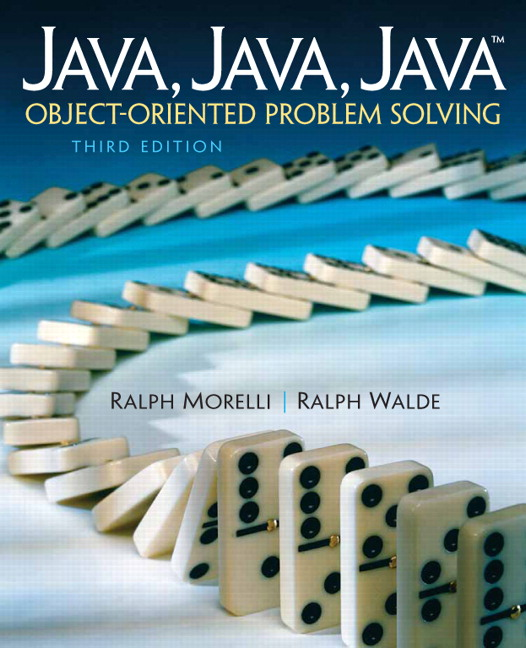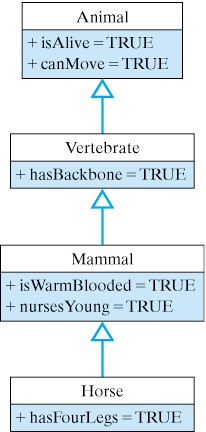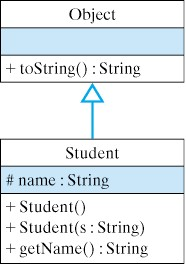# Java, Java, Java: Object-Oriented Problem Solving, 2022E

## Section8.2Java’s Inheritance Mechanism

As we described in Chapter 0, class inheritance is the mechanism whereby a class acquires (inherits) the methods and variables of its superclasses. To remind you of the basic concept, let’s repeat an earlier example: Just as horses inherit the attributes and behaviors associated with mammals and vertebrates, a Java subclass inherits the attributes and behaviors of its superclasses.Figure 8.2.1. Figure 8.2.1 uses a UML diagram to illustrate the relationships among horses, mammals, vertebrates, and animals. As the root of the hierarchy, which is always shown at the top, the Animal class contains the most general attributes, such as being alive and being able to move. All animals share these attributes. The class of vertebrates is a somewhat more specialized type of animal, in that vertebrates have backbones. Similarly, the class of mammals is a further specialization over the vertebrates in that mammals are warm-blooded and nurse their young. Finally, the class of horses is a further specialization over the class of mammals, in that all horses have four legs, but it inherits the features of the classes above it.

### Subsection8.2.1Using an Inherited Method

In Java, the public and protected instance methods and instance variables of a superclass are inherited by all of its subclasses. This means that objects belonging to the subclasses can use the inherited variables and methods as their own.
We have already seen some examples of this in earlier chapters. For example, recall that by default all Java classes are subclasses of the Object class, which is the most general class in Java’s class hierarchy. One public method that is defined in the Object class is the toString() method. Because every class in the Java hierarchy is a subclass of Object, every class inherits the toString() method. Therefore, toString() can be used with any Java object.
To illustrate this, suppose we define a Student class as follows:
public class Student {
protected String name;
public Student(String s) {
name = s;
}
public String getName() {
return name;
}
}

Figure 8.2.2 shows the relationship between this class and the Object class.Figure 8.2.2. Student inherits from Object As a subclass of Object, the Student class inherits the toString() method. Therefore, for a given Student object, we can call its toString() as follows:
Student stu = new Student("Stu");
System.out.println(stu.toString());

How does Java know where to find the toString() method, which, after all, is not defined in the Student class? When the expression stu.toString() is executed, Java will first look in the Student class for a definition of the toString() method. Not finding one there, it will then search up the Student class hierarchy () until it finds a public or protected definition of the toString() method. In this case, it finds a toString() method in the Object class and it executes that implementation of toString(). As you know from Chapter 3, this would result in the expression stu.toString() returning something like: Student@cde100.
The default implementation of toString() returns the name of the object’s class and the address (cde100) where the object is stored in memory. However, this type of result is much too general and not particularly useful. See it running below.

#### Activity8.2.1.

Run to see the result of the Object’s toString() method below.

### Subsection8.2.2Overriding an Inherited Method

In Chapter 3 we pointed out that the toString() method is designed to be overridden—that is, to be redefined in subclasses of Object. Overriding toString() in a subclass provides a customized string representation of the objects in that subclass. We showed that by redefining toString() in our OneRowNim class, we customized its actions so that it returned useful information about the current state of a OneRowNim game.
To override toString() for the Student class, let’s add the following method definition to the Student class:
public String toString() {
return "My name is " + name +  " and I am a Student.";
}

Given this change, the revised Student class hierarchy is shown in Figure 8.2.3. Note that both Object and Student contain implementations of toString(). Now when the expression stu.toString() is invoked, the following, more informative, output is generated: My name is Stu and I am a Student..
In this case, when Java encounters the method call stu.toString(), it invokes the toString() method that it finds in the Student class. Run this code below.

#### Activity8.2.2.

Run to see the result of the overriden toString() method below.
These examples illustrate two important object-oriented concepts: inheritance and method overriding.

### Subsection8.2.3Static Binding, Dynamic Binding and Polymorphism

The mechanism that Java uses in these examples is known as dynamic binding, in which the association between a method call and the correct method implementation is made at run time. In dynamic binding a method call is bound to the correct implementation of the method at run time by the Java Virtual Machine (JVM).
Dynamic binding is contrasted with static binding, the mechanism by which the Java compiler resolves the association between a method call and the correct method implementation when the program is compiled. In dynamic binding, when the JVM encounters a method call, it uses information about the class hierarchy to bind the method call to the correct implementation of that method.
In Java, all method calls use dynamic binding except methods that are declared final or private. Final methods cannot be overridden, so declaring a method as final means that the Java compiler can bind it to the correct implementation. Similarly, private methods are not inherited and therefore cannot be overridden in a subclass. In effect, private methods are final methods and the compiler can perform the binding at compile time.
Java’s dynamic-binding mechanism, which is also called late binding or run-time binding, leads to what is know as polymorphism. Polymorphism is a feature of object-oriented languages whereby the same method call can lead to different behaviors depending on the type of object on which the method call is made. The term polymorphism means, literally, having many (poly) shapes (morphs). Here’s a simple example:
Object obj;                        // Static type: Object
obj = new Student("Stu");          // Actual type: Student
System.out.println(obj.toString());// Prints "My name is Stu..."
obj = new OneRowNim(11);           // Actual type: OneRowNim
System.out.println(obj.toString());// Prints "nSticks = 11, player = 1"

The variable obj is declared to be of type Object. This is its static or declared type. A variable’s static type never changes. However, a variable also has an actual or dynamic type. This is the actual type of the object that has been assigned to the variable. As you know, an Object variable can be assigned objects from any Object subclass. In the second statement, obj is assigned a Student object. Thus, at this point in the program, the actual type of the variable obj is Student. When obj.toString() is invoked in the third line, Java begins its search for the toString() method at the Student class, because that is the variable’s actual type.
In the fourth line, we assign a OneRowNim object to obj, thereby changing its actual type to OneRowNim. Thus, when obj.toString() is invoked in the last line, the toString() method is bound to the implementation found in the OneRowNim class.
Thus, we see that the same expression, obj.toString(), is bound alternatively to two different toString() implementations, based on the actual type of the object, obj, on which it is invoked. This is polymorphism and we will sometimes say that the  toString() method is a polymorphic method. A polymorphic method is a method signature that behaves differently when it is invoked on different objects. An overridden method, such as the toString() method, is an example of a polymorphic method, because its use can lead to different behaviors depending upon the object on which it is invoked.
Let’s take an example where static binding, also called early binding, is not possible. Consider the following method definition:
public void polyMethod(Object obj) {
System.out.println(obj.toString()); // Polymorphic
}

The method call in this method, obj.toString(), can’t be bound to the correct implementation of toString() until the method is actually invoked—that is, at run time. For example, suppose we make the following method calls in a program:
Student stu = new Student("Stu");
polyMethod(stu);
OneRowNim nim = new OneRowNim();
polyMethod(nim);

The first time polyMethod() is called, the obj.toString() is invoked on a Student object. Java will use its dynamic binding mechanism to associate this method call with the toString() implementation in Student and output “My name is Stu and I am a Student.” The second time polyMethod() is called, the obj.toString() expression is invoked on a OneRowNim object. In this case, Java will bind the method call to the implementation in the OneRowNim class. The output generated in this case will report how many sticks are left in the game.
The important point here is that polymorphism occurs when an overridden method is called on a superclass variable, obj. In such a case, the actual method implementation that is invoked is determined at run time. The determination depends on the type of object that was assigned to the variable. Thus, we say that the method call obj.toString() is polymorphic because it is bound to different implementations of toString() depending on the actual type of the object that is bound to obj.

### Subsection8.2.4Polymorphism and Object-Oriented Design

Now that we understand how inheritance and polymorphism work in Java, it will be useful to consider an example that illustrates how these mechanisms can be useful in designing classes and methods. We have been using the various System.out.print() and System.out.println() methods since Chapter 1. The print() and println() methods are examples of overloaded methods—that is, methods that have the same name but different parameter lists. Remember that a method’s signature involves its name, plus the type, number, and order of its parameters. Methods that have the same name but different parameters are said to be overloaded.
Here are the signatures of some of the different print() and println() methods:
print(char c);           println(char c);
print(int i);            println(int i);
print(double d);         println(double d);
print(float f);          println(float f);
print(String s);         println(String s);
print(Object o);         println(Object o);

Basically, there is a print() and println() method for every type of primitive data, plus methods for printing any type of object. When Java encounters an expression involving print() or println() it chooses which particular print() or println() method to call. To determine the correct method, Java relies on the differences in the signatures of the various print() methods. For example, because its argument is an int, the expression print(5) is associated with the method whose signature is print(int i) be cause its parameter is an int.
Note that there is only one set of print() and println() methods for printing Object s. The reason is that polymorphism is used by the print(Object o) and println(Object o) methods to print any type of object. While we do not have access to the source code for these methods, we can make an educated guess that their implementations utilize the polymorphic toString() method, as follows:
public void print(Object o) {
System.out.print(o.toString());
}
public void println(Object o) {
System.out.println(o.toString());
}

Here again we have a case where an expression, o.toString(), is bound dynamically to the correct implementation of toString() based on the type of Object that the variable o is bound to. If we call System.out.print(stu), where stu is a Student, then the Student.toString() method is invoked. On the other hand, if we call System.out.print(game), where game is a OneRowNim, then the OneRowNim.toString() method is invoked.
The beauty of using polymorphism in this way is the flexibility and extensibility that it allows. The print() and println() methods can print any type of object, even new types of objects that did not exist when these library methods were written.

#### ExercisesSelf-Study Exercises

##### 1.
Run the TestPrint program below. Override the toString() method in the TestPrint class and rerun. Add a comment describing how it confirms how print() and println() methods are implemented.

### Subsection8.2.5Using super to Refer to the Superclass

One question that might occur to you is: Once you override the default toString() method, is it then impossible to invoke the default method on a Student object? The default toString() method (and any method from an object’s superclass) can be invoked using the super keyword. For example, suppose that within the Student class, you wanted to concatenate the result of both the default and the new toString() methods. The following expression would accomplish that:
super.toString() + toString()

The super keyword specifies that the first toString() is the one implemented in the superclass. The second toString() refers simply to the version implemented within the Student class. We will see additional examples of using the super keyword in the following sections.

#### ExercisesSelf-Study Exercises

##### 1.B subclasses A.
Given the following class definitions, what would be output by the code segment?
Hint.
Try running the code with codelens.
##### 2.B subclasses A, Part 2.
Modify class B’s method() so that it invokes A’s version of method() before printing out B. What would be output in this case?
Hint.
Try running the code with codelens. Use super.method().
##### 3.Which are valid?
Given the definitions of the classes A and B in the previous exercises, such that B is a subclass of A, which of the following statements are valid?
A a = new B();
a = new A();
B b = new A();
b = new B();

• A a = new B();
• B is a subclass of A so this is valid.
• a = new A();
• The variable a has type A.
• B b = new A();
• A is a superclass of B so this is not valid.
• b = new B();
• The variable a has type B.

### Subsection8.2.6Inheritance and Constructors

Java’s inheritance mechanism applies to a class’s public and protected instance variables and methods. It does not apply to a class’s constructors. To illustrate some of the implications of this language feature, let’s define a subclass of Student called CollegeStudent:
public class CollegeStudent extends Student {
public CollegeStudent() { }
public CollegeStudent(String s) {
super(s);
}
public String toString() {
return "My name is " + name +
" and I am a CollegeStudent.";
}
}

Because CollegeStudent is a subclass of Student, it inherits the public and protected instance methods and variables from Student. So, a CollegeStudent has an instance variable for name and it has a public getName() method.
Recall that a protected element, such as the name variable in the Student class, is accessible only within the class and its subclasses. Unlike public elements, it is not accessible to other classes.
Note that CollegeStudent overrides the toString() method, giving it a more customized implementation. The hierarchical relationship between CollegeStudent and Student is shown in Figure 8.2.6. A CollegeStudent is a Student and both are Objects.
Note how we have implemented the CollegeStudent(String s) constructor. Because the superclass’s constructors are not inherited, we have to implement this constructor in the subclass if we want to be able to assign a CollegeStudent’s name during object construction. The method call, super(s), is used to invoke the superclass constructor and pass it s, the student’s name. The superclass constructor will then assign s to the name variable.
As we have noted, a subclass does not inherit constructors from its superclasses. However, if the subclass constructor does not explicitly invoke a superclass constructor, Java will automatically invoke the default superclass constructor—in this case, super(). By default superclass constructor we mean the constructor that has no parameters. For a subclass that is several layers down in the hierarchy, this automatic invoking of the super() constructor will be repeated upwards through the entire class hierarchy. Thus when a CollegeStudent is constructed, Java will automatically call Student() and Object(). Note that if one of the superclasses does not contain a default constructor, this will result in a syntax error.
If you think about this, it makes good sense. How else will the inherited elements of the object be created? For example, in order for a CollegeStudent to have a name variable, a Student object, where name is declared, must be created. The CollegeStudent constructor then extends the definition of the Student class. Similarly, in order for a Student object to have the attributes common to all objects, an Object instance must be created and then extended into a Student.
Thus, unless a constructor explicitly calls a superclass constructor, Java will automatically invoke the default superclass constructors. It does this before executing the code in its own constructor.
Calls to the default constructors are made all the way up the class hierarchy, and the superclass constructor is always called before the code in the class’s constructor is executed.

#### ExercisesSelf-Study Exercises

##### 1.Super Constructors.
Given the following class definitions, what would be output by the code segment?
public class A {
public A() { System.out.print("A"); }
}
public class B extends A {
public B() { System.out.print("B"); }
}
public class C extends B {
public C() { System.out.print("C"); }
}
// Determine the output.
A a = new A();
B b = new B();
C c = new C();

Hint.
Try running the code with codelens.